# Proportion Worksheet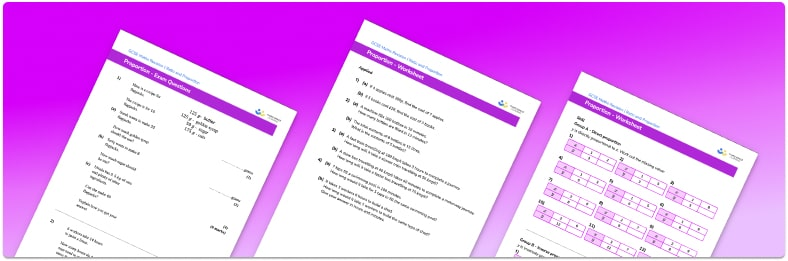• This field is for validation purposes and should be left unchanged.

You can unsubscribe at any time (each email we send will contain an easy way to unsubscribe). To find out more about how we use your data, see our privacy policy.

• Section 1 of the proportion worksheet contains 36 skills-based proportion questions, in 3 groups to support differentiation
• Section 2 contains 4 applied proportion questions with a mix of worded problems and deeper problem solving questions
• Section 3 contains 4 foundation and higher level GCSE exam style ratio scale questions
• Answers and a mark scheme for all ratio scale questions are provided
• Questions follow variation theory with plenty of opportunities for students to work independently at their own level
• All questions created by fully qualified expert secondary maths teachers
• Suitable for GCSE maths revision for AQA, OCR and Edexcel exam boards

### Proportion at a glance

If two amounts are proportional then the ratio between them is always the same. Proportional relationships can be direct or inverse. In direct proportion, as one amount increases so does the other whereas in inverse proportion, as one amount increases the other decreases.

Given some information about a proportional relationship, we can find the constant of proportionality. This tells us how many times bigger one amount is than the other. This value will always be true for the given relationship.

If y is directly proportional to x then y is equal to k times x where k is the constant of proportionality.

If y is inversely proportional to x then y is equal to k divided by x where k is the constant of proportionality.

Looking forward, students can then progress to additional ratio and proportion worksheets, for example aor a ratio worksheet.

For more teaching and learning support on Ratio and Proportion our GCSE maths lessons provide step by step support for all GCSE maths concepts.

## Related worksheets

Inverse Proportion Worksheet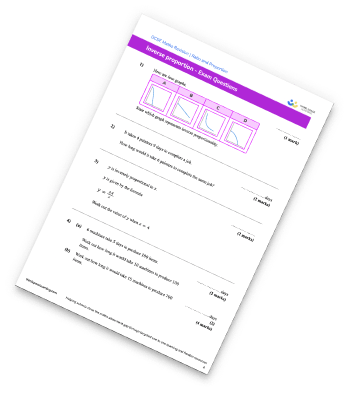Direct Proportion Worksheet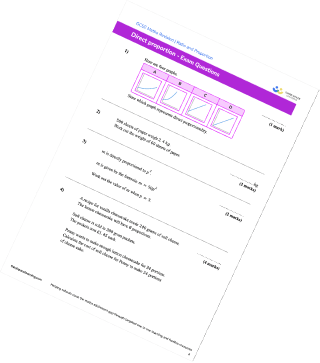Directly Proportional Graph/ Inversely Proportional Graph Worksheet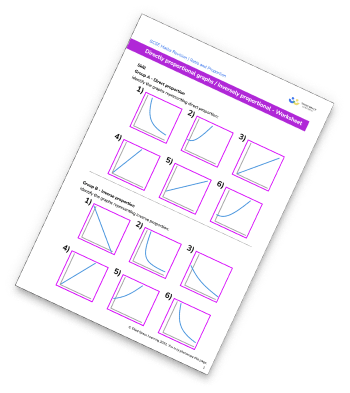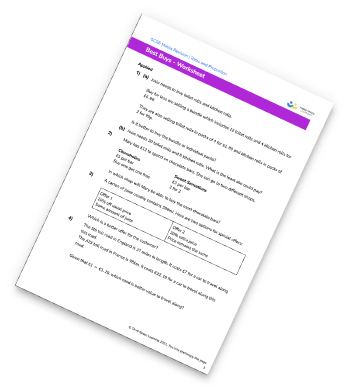## Do you have KS4 students who need more focused attention to succeed at GCSE?There will be students in your class who require individual attention to help them succeed in their maths GCSEs. In a class of 30, it’s not always easy to provide.

Help your students feel confident with exam-style questions and the strategies they’ll need to answer them correctly with our dedicated GCSE maths revision programme.

Lessons are selected to provide support where each student needs it most, and specially-trained GCSE maths tutors adapt the pitch and pace of each lesson. This ensures a personalised revision programme that raises grades and boosts confidence.

Find out more